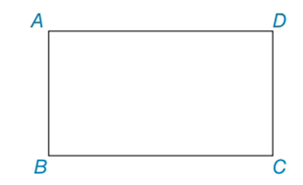Chapter 4.3, Problem 10EElementary Geometry For College St...

7th Edition
Alexander + 2 others
ISBN: 9781337614085

Solutions

Chapter
SectionElementary Geometry For College St...

7th Edition
Alexander + 2 others
ISBN: 9781337614085
Textbook Problem

In Exercises 8 to 10, use the properties of rectangles to solve each problem. Rectangle ABCD is shown in the figure.Exercises 8 – 10 Given: AB = x + y and BC = x + 2y, and CD = 2x – y – 1, and DA = 3x – 3y + 1 Find: x and y

To determine

To find:

x and y of the given rectangle.

Explanation

The basic property of rectangle:

1) All the properties of a parallelogram apply (the ones that matter here are parallel sides, opposite sides are congruent, and diagonals bisect each other).

2) All angles are right angles by definition.

3) The diagonals are congruent.

Calculation:

Given: AB = x + y and BC = x + 2y, and CD = 2xy – 1, and DA = 3x – 3y + 1

Consider the rectangle.

Now we have to find x and y of the given rectangle.

To find x and y:

From the property, opposite sides are congruent in rectangle.

So, AB = CD

x+y=2xy1(1)

BC = DA

x+2y=3x3y+1(2)

Now we have to solve (1) and (2).

By solving (1), we get

x+y=2xy12xx=y+y+1x=2y+1

By solving (2), we get

x+2y=3x3y+

Still sussing out bartleby?

Check out a sample textbook solution.

See a sample solution

The Solution to Your Study Problems

Bartleby provides explanations to thousands of textbook problems written by our experts, many with advanced degrees!

Get Started

Justify 3 for the case h0.

Calculus (MindTap Course List)

In Exercises 4144, determine whether the statement is true or false. 44. 561112

Applied Calculus for the Managerial, Life, and Social Sciences: A Brief Approach

Rewritten as an iterated integral in polar coordinates,

Study Guide for Stewart's Multivariable Calculus, 8th

In the graph at the right, limx1f(x)=. a) 2 b) 1 c) 3 d) does not exist

Study Guide for Stewart's Single Variable Calculus: Early Transcendentals, 8th